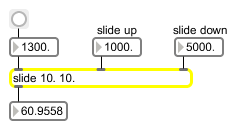# slide

Filter an input value logarithmically

## Description

slide filters an input value logarithmically between changes. The formula is y (n) = y (n-1) + ((x (n) - y (n-1))/slide). It's particularly useful for envelope following and lowpass filtering.

## Arguments

Name Type Opt Description
slide-up-value float opt Specifies the slide up value. The default is 1.
slide-down-value float opt A second argument specifies the slide down value. The default is 1.

## Messages

 bang Performs the same function as float using the last input value. int input [int] Converted to float. float input [float] In left inlet: An input value to be filtered. The a new value is received, object filters an input value logarithmically between changes using the formula y (n) = y (n-1) + ((x (n) - y (n-1))/slide) A given sample output from slide is equal to the last value plus the difference between the last value and the input divided by the slide value. Given a slide value of 1, the output will therefore always equal the input. Given a slide value of 10, the output will only change 1/10th as quickly as the input. This can be particularly useful for lowpass filtering or envelope following. (inlet1) slide-up-value [float] In middle inlet: Specifies the slide up value to be used when an incoming value is greater than the current value. (inlet2) slide-down-value [float] In right inlet: Specifies the slide down value to be used when an incoming value is less than the current value. set input [int] The word set followed by a number will set the current input value to the given number without causing output (bang can be used to cause successive output). reset Resets the current output sample to 0.

## Output

float: The filtered input value.

## Examplesslide performs logarithmic smoothing of an input